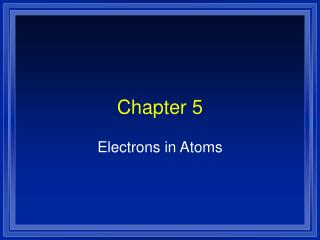DownloadDownload PresentationChapter 5

# Chapter 5

Download Presentation## Chapter 5

- - - - - - - - - - - - - - - - - - - - - - - - - - - E N D - - - - - - - - - - - - - - - - - - - - - - - - - - -
##### Presentation Transcript

1. Chapter 5 Electrons in Atoms

2. Light and Quantized Energy (5.1) • The study of light led to the development of the quantum mechanical model. • Light is a kind of electromagnetic radiation EM). • All move at 3.00 x 108 m/s (c) Speed of light.

3. Crest Wavelength Amplitude Trough Parts of a wave Origin

4. Parts of Wave • Crest - high point on a wave • Trough - Low point on a wave • Amplitude - distance from origin to crest • Wavelength (l) - distance from crest to crest. To calculate use: l=c/v. • c = speed of light (3.00 x 108 m/s). • V = frequency (HZ)

5. Frequency • Frequency (v) is the number of waves that pass a given point per second. Units are cycles/sec or hertz (Hz). To calculate use: • v = c/l

6. Frequency and wavelength • Are inversely related (v = c/l ) • As one goes up the other goes down. • Different frequencies of light show as different colors of light. • The whole range is called the electromagnetic (EM) spectrum

7. High energy Low energy Low Frequency High Frequency Spectrum X-Rays Radio waves Microwaves Ultra-violet Gamma Rays Infrared . Long Wavelength Short Wavelength Visible Light

8. Light is a Particle • Light is energy, Energy is quantized, therefore, Light must be quantized. • These quantized pieces of light are called photons. • Energy and frequency of the photons are directly related. E = h x n • (i.e.. High frequency = high energy)

9. Energy and frequency • A photon is a particle of EM radiation with no mass that carries a quantum of energy. To calculate its energy use: • EPhoton = h x n E is the energy of the photon nis the frequency h is Planck’s constant (6.626 x 10 -34 Joules sec).

10. Photoelectric Effect • In the photoelectriceffect , electrons, called photoelectrons, are emitted from a metals surface when light of a certain frequency shines on it. (solar calculator) • Can be used to identify the type of metal.

11. Examples • What is the frequency of red light with a wavelength of 4.2 x 10-5 cm? • What is the wavelength of KFI, which broadcasts at with a frequency of 640 kHz? • What is the energy of a photon of each of the above?

12. Atomic Emission Spectrum How color tells us about atoms? The atomic emission spectrum of an element is the set of frequencies of the EM waves emitted by atoms of the element. Each is unique to the individual element giving a pattern of visible colors when viewed through a prism.

13. Prism • White light is made up of all the colors of the visible spectrum. • Passing it through a prism separates it into colors.

14. If the light is not white • By heating a gas or with electricity we can get it to give off colors. • Passing this light through a prism shows a unique color pattern

15. Atomic Emission Spectrum • Each element gives off its own characteristic colors. • Can be used to identify the atom. • This is how we know what stars are made of.

16. These are called line spectra • unique to each element. • These are emission spectra • Mirror images are absorption spectra • Light with black missing

17. An explanation of the Atomic Emission Spectra

18. Where the electron starts • When we write electron configurations we are starting at the writing the lowest energy level. • The energy level an electron starts from is called its groundstate.

19. Changing the energy • Let’s look at a hydrogen atom

20. Changing the energy • Heat or electricity or light can move the electron up energy levels

21. Changing the energy • As the electron falls back to ground state it gives the energy back as light

22. Changing the energy • May fall down in steps • Each with a different energy

23. The Bohr Ring Atom n = 4 n = 3 n = 2 n = 1

24. { { {

25. The Further the electrons fall, the more the energy and the higher the frequency. Ultraviolet Visible Infrared

26. Light is also a wave • Light is a particle - it comes in chunks. • Light is also a wave- we can measure its wave length and it behaves as a wave • The wavelength of a particle is calculated using l = h/mv . (de Broglie equation)

27. Diffraction • When light passes through, or reflects off, a series of thinly spaced lines, it creates a rainbow effect because the waves interfere with each other.

28. A wave moves toward a slit.

29. A wave moves toward a slit.

30. A wave moves toward a slit.

31. A wave moves toward a slit.

32. A wave moves toward a slit.

33. Comes out as a curve

34. Comes out as a curve

35. Comes out as a curve

36. with two holes

37. with two holes

38. with two holes

39. with two holes

40. with two holes

41. Two Curves with two holes

42. Two Curves with two holes

43. Two Curves with two holes Interfere with each other

44. Two Curves with two holes Interfere with each other crests add up

45. Several waves

46. Several waves

47. Several waves

48. Several waves